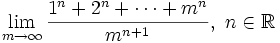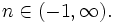# Solution July 26, 2007

Solution by landen

Find the following limit in terms of$n\,.$$\lim_{m\rightarrow \infty} \frac{1^n+2^n+\dots+m^n}{m^{n+1}},\ n \in \mathbb{R}$ with$n \in (-1,\infty).\,$

The plan is to use a Riemann sum (http://en.wikipedia.org/wiki/Riemann_integral) to convert the limit into an integral.$\lim_{m\rightarrow \infty} \frac{1^n+2^n+\dots+m^n}{m^{n+1}} = \lim_{m\rightarrow \infty} \frac{1}{m}\sum_{j=1}^m \left(\frac{j}{m}\right)^n=\int_0^1 x^n dx=\frac{1}{n+1}$